# 32 Results

View
Selected filters:
• MI.Math.Content.8.EE.B.5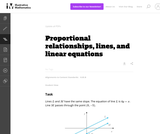Unrestricted Use
CC BY
Rating

This is a task from the Illustrative Mathematics website that is one part of a complete illustration of the standard to which it is aligned. Each task has at least one solution and some commentary that addresses important asects of the task and its potential use. Here are the first few lines of the commentary for this task: Lines \$L\$ and \$M\$ have the same slope. The equation of line \$L\$ is \$4y=x\$. Line \$M\$ passes through the point \$(0, -5)\$. What is the equation of line \$M...

Subject:
Mathematics
Material Type:
Activity/Lab
Provider:
Illustrative Mathematics
Provider Set:
Illustrative Mathematics
Author:
Illustrative Mathematics
05/28/2013Unrestricted Use
CC BY
Rating

This is a task from the Illustrative Mathematics website that is one part of a complete illustration of the standard to which it is aligned. Each task has at least one solution and some commentary that addresses important asects of the task and its potential use. Here are the first few lines of the commentary for this task: Anna and Jason have summer jobs stuffing envelopes for two different companies. Anna earns \\$14 for every 400 envelops she finishes. Jason earns \\$9 fo...

Subject:
Mathematics
Material Type:
Activity/Lab
Provider:
Illustrative Mathematics
Provider Set:
Illustrative Mathematics
Author:
Illustrative Mathematics
08/12/2013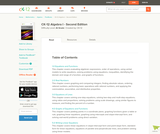Conditional Remix & Share Permitted
CC BY-NC-SA
Rating

A work in progress, CK-12's Algebra I Second Edition is a clear presentation of algebra for the high school student. Topics include: Equations and Functions, Real Numbers, Equations of Lines, Solving Systems of Equations and Quadratic Equations.

Subject:
Algebra
Functions
Material Type:
Textbook
Provider:
CK-12 Foundation
Provider Set:
CK-12 FlexBook
Author:
Andrew
Annamaria
Anne
Eve
Farbizio
Gloag
Rawley
12/03/2010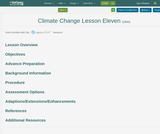Conditional Remix & Share Permitted
CC BY-NC
Rating

Climagraphs can tell us about the seasonal shifts in climate due to climate change. Changes in growing season and water balance in the Great Lakes region will have economic impacts.

Subject:
Environmental Science
Material Type:
Activity/Lab
Lesson Plan
Author:
Michigan Geographic Alliance
08/28/2020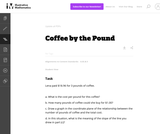Unrestricted Use
CC BY
Rating

Students are exptected to identify the slope of the line with the unit rate in this real world problem.

Subject:
Mathematics
Material Type:
Activity/Lab
Provider:
Illustrative Mathematics
Provider Set:
Illustrative Mathematics
Author:
Illustrative Mathematics
05/01/2012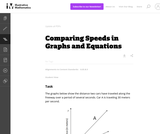Unrestricted Use
CC BY
Rating

This task provides the opportunity for students to reason about graphs, slopes, and rates without having a scale on the axes or an equation to represent the graphs. Students who prefer to work with specific numbers can write in scales on the axes to help them get started.

Subject:
Mathematics
Material Type:
Activity/Lab
Provider:
Illustrative Mathematics
Provider Set:
Illustrative Mathematics
Author:
Illustrative Mathematics
05/01/2012Unrestricted Use
CC BY
Rating

This task requires students to work with unit rates.

Subject:
Mathematics
Material Type:
Activity/Lab
Provider:
Illustrative Mathematics
Provider Set:
Illustrative Mathematics
Author:
Illustrative Mathematics
05/01/2012Conditional Remix & Share Permitted
CC BY-NC-SA
Rating

An interactive applet and associated web page that demonstrate the equation of a line in point-slope form. The user can move a slider that controls the slope, and can drag the point that defines the line. The graph changes accordingly and equation for the line is continuously recalculated with every slider and / or point move. The grid, axis pointers and coordinates can be turned on and off. The equation display can be turned off to permit class exercises and then turned back on the verify the answers. The applet can be printed as it appears on the screen to make handouts. The web page has a full description of the concept of the equation of a line in point - slope form, a worked example and has links to other pages relating to coordinate geometry. Applet can be enlarged to full screen size for use with a classroom projector. This resource is a component of the Math Open Reference Interactive Geometry textbook project at http://www.mathopenref.com.

Subject:
Mathematics
Material Type:
Simulation
Author:
John Page
08/05/2020Unrestricted Use
CC BY
Rating

This task requires students to use the fact that on the graph of the linear equation y=ax+c, the y-coordinate increases by a when x increases by one. Specific values for c and d were left out intentionally to encourage students to use the above fact as opposed to computing the point of intersection, (p,q), and then computing respective function values to answer the question.

Subject:
Mathematics
Material Type:
Activity/Lab
Provider:
Illustrative Mathematics
Provider Set:
Illustrative Mathematics
Author:
Illustrative Mathematics
05/01/2012Unrestricted Use
CC BY
Rating

This task helps students solidify their understanding of linear functions and push them to be more fluent in their reasoning about slope and y-intercepts. This task has also produced a reasonable starting place for discussing point-slope form of a linear equation.

Subject:
Mathematics
Material Type:
Activity/Lab
Provider:
Illustrative Mathematics
Provider Set:
Illustrative Mathematics
Author:
Illustrative Mathematics
05/01/2012Conditional Remix & Share Permitted
CC BY-NC-SA
Rating

In this video segment from Cyberchase, Harry decides to train as a firefighter and uses line graphs to chart his physical fitness progress.

Subject:
Mathematics
Physics
Material Type:
Lecture
Author:
U.S. Department of Education
WNET
08/05/2020Only Sharing Permitted
CC BY-NC-ND
Rating

This lesson unit is intended to help you assess how well students working with square numbers are able to: choose an appropriate, systematic way to collect and organize data, examining the data for patterns; describe and explain findings clearly and effectively; generalize using numerical, geometrical, graphical and/or algebraic structure; and explain why certain results are possible/impossible, moving towards a proof.

Subject:
Algebra
Geometry
Measurement and Data
Material Type:
Assessment
Lesson Plan
Author:
Shell Center for Mathematical Education
U.C. Berkeley
08/10/2020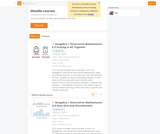Conditional Remix & Share Permitted
CC BY-NC
Rating

This link takes you to MoodleNet where you can use and download Middle School level math lessons that use both Geogebra and Illustrative Math.

Subject:
Mathematics
Material Type:
Activity/Lab
Assessment
Homework/Assignment
02/22/2021Conditional Remix & Share Permitted
CC BY-NC-SA
Rating

In Module 4, students extend what they already know about unit rates and proportional relationships to linear equations and their graphs.  Students understand the connections between proportional relationships, lines, and linear equations in this module.  Students learn to apply the skills they acquired in Grades 6 and 7, with respect to symbolic notation and properties of equality to transcribe and solve equations in one variable and then in two variables.

Subject:
Mathematics
Material Type:
Module
Author:
EngageNY
New York State Education Department
08/06/2020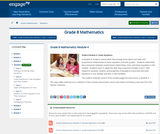Conditional Remix & Share Permitted
CC BY-NC-SA
Rating

In Module 4, students extend what they already know about unit rates and proportional relationships to linear equations and their graphs.  Students understand the connections between proportional relationships, lines, and linear equations in this module.  Students learn to apply the skills they acquired in Grades 6 and 7, with respect to symbolic notation and properties of equality to transcribe and solve equations in one variable and then in two variables.

Subject:
Algebra
Material Type:
Module
Provider:
New York State Education Department
Provider Set:
EngageNY
11/12/2013Unrestricted Use
CC BY
Rating

Student-facing 8th grade math resources. Covers transformations & congruence, slope, similarity, linear relationships, associations in data, volume, functions, scientific notation, the pythagorean theorem and irrational numbers.

Subject:
Mathematics
Material Type:
Unit of Study
Author:
Open Up Resources Illustrative Mathematics
08/07/2020Conditional Remix & Share Permitted
CC BY-NC-SA
Rating

In this lesson designed to enhance literacy skills, students learn how to read and interpret a distance&ndash;time graph.

Subject:
Mathematics
Material Type:
Activity/Lab
Author:
PBS LearningMedia
08/04/2020Rating

Students learn about an important characteristic of lines: their slopes. Slope can be determined either in graphical or algebraic form. Slope can also be described as positive, negative, zero or undefined. Students get an explanation of when and how these different types of slope occur. Finally, they learn how slope relates to parallel and perpendicular lines. When two lines are parallel, they have the same slope and when they are perpendicular their slopes are negative reciprocals of one another.

Subject:
Mathematics
Material Type:
Homework/Assignment
Lesson Plan
Author:
Aubrey Mckelvey
08/06/2020Conditional Remix & Share Permitted
CC BY-NC-SA
Rating

Students will use ratios to demonstrate the connections between proportional relationships, lines, and linear equations. Students will solve problems using a cooperative, kinesthetic activity in which they will create a ratio table, then graph proportional relationships with their bodies to demonstrate that the ratio (or rate) is the slope that will always pass through the origin.

Subject:
Mathematics
Material Type:
Activity/Lab
Lesson Plan
Teaching/Learning Strategy
Author:
Pat C. Browne
08/06/2020Only Sharing Permitted
CC BY-NC-ND
Rating

This lesson unit is intended to help teahcers assess how well students are able to interpret speed as the slope of a linear graph and translate between the equation of a line and its graphical representation.

Subject:
Mathematics
Material Type:
Assessment
Lesson Plan
Author:
Mathematics Assessment Project (MAP)
Shell Center for Mathematical Education